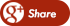﻿ what is MIMO?Back

## what is MIMO?

By Admin onWhat is MIMO-The full form of MIMO is multi input and multi output where signals are transmitted via multiple antennas instead of only one antenna like FDM. This technique has the potential of dramatic increase of data transmission in wireless environment.What is OFDM- it is a combination of both modulation and multiplexing. Unlike general multiplexing technique it is applied to only one independent channel, where a set of signals from the same source is divided or spitted into a number of independent channels (sub-set of the main signals) and each modulated by separate carrier, and then multiplexed into an OFDM signal for transmission. Each independent sub-set channel can be multiplexed either by frequency or code division multiplexing.

Characteristics of OFDM sub-channels

As I already mentioned that OFDM is a special set of FDM, but what makes it special is the orthogonality of the sub-carries. That means each sub-channel of the main signal in fact multiplied by a carrier which is orthogonal to each other. Carriers are nothing but sine or cosine wave; and the area under one sine and one cosine wave is simply zero. Orthogonal carrier means if the first carrier frequency is f1 the second carrier will be 2xf1, the third carrier will be 3xf1, and so on. This kind of orthogonality of the carriers allows simultaneous transmission of sub-carriers in a tightly spaced bandwidth without interfering from each other because each carrier is orthogonal to each other making the result of their multiplication a zero. Normally, OFDM signals are sent via one transmitting antenna.

Multiplexing-mulplexing is a method of sharing bandwidth with some other independent communication data channels.

MIMO-OFDM definition-when generated OFDM signal is transmitted through a number of antennas in order to achieve diversity or cap any gain (higher transmission rate) then it is known as MIMO-OFDMHow MIMO-OFDM Works? Like any other communication systems MIMO-OFDM system also has transmitter and receiver, but it has multiple antennas -both at transmit and receive end. MIMO system can be implemented in various ways. If we need to take the diversity as an advantage to combat fading then we need to send the same signals through various MIMO antennas and at the receiving end all the signals received by MIMO antennas will receive the same signals traveled through various path. In this case the entire received signal must pass through un-correlated channels. If we are inserted to use MIMO for capacity increase then we can send different set of data (not the same set of data like diversity MIMO) via a number of antennas and the same number of antennas will receive the signals in the receiving end. For MIMO to be efficient antenna spacing need to be done very carefully- at least half the wavelength of the transmitting signal.

Advantage of MIMO-OFDM

• Less interference
• Diversity gain
• Increase data capacity
• Power efficiency
• Bandwidth gain

MIMO_OFDM research Universities

• University of Southampton
• Rich University
• University of York
• University of Maryland
• National Tsing Hua University
• Korean Institute of Advanced Science and Technology

Limitations of MIMO-OFDM

• Antenna spacing must be appropriate depending on the type of channels
• Very complex transmitter and receiver

MIMO-OFDM Research ideas

• Application of CMDA in MIMO-OFDM
• OFDMA
• Adaptive MIMO antenna selection algorithms.
• Frequency hopping in the presence of frequency selective channels.
• Noise torelance and interference cancellation
• MIMO channel estimation technique developments

FOR MORE DETAILS CLICK HERE